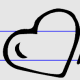Home > Nanette Johnson (page 5)

## Nanette Johnson## System of Equations, Special Case Infinitely Many Solutions

Directions: Using the digits 1 to 9 at most one time each, fill in the boxes so that there are infinitely many solutions to the system of equations. Source: Nanette Johnson

## Systems of Equations, Special Case No Solution

Directions: Using the digits 1 to 9 at most one time each, fill in the boxes so that there is no solution to the system of equations. Source: Nanette Johnson

## Solve Linear Equations with Special Cases

Source: SBAC Practice Test 8th Grade

Source: Smarter Balanced Practice Question

Directions: Fill in the blanks with numbers that make the equation true. 4 + 5 = ___ – ___   Source: Nanette Johnson

## Square Circumscribed about a Circle

Directions: What is the area of the smallest square that could fit around (circumscribed about) a circle with area 100π square units? Source: Nanette Johnson

## Quadrilateral Inscribed in a Circle

Directions: What is the biggest area of a quadrilateral you can fit in a circle that has a circumference of 20π units? Source: Nanette Johnson